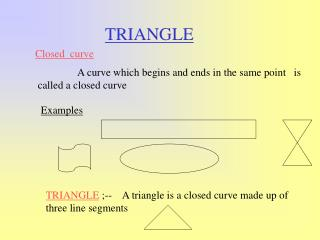DownloadDownload PresentationTRIANGLE

# TRIANGLE

Télécharger la présentation## TRIANGLE

- - - - - - - - - - - - - - - - - - - - - - - - - - - E N D - - - - - - - - - - - - - - - - - - - - - - - - - - -
##### Presentation Transcript

1. TRIANGLE Closed curve A curve which begins and ends in the same point is called a closed curve Examples TRIANGLE ;-- A triangle is a closed curve made up of three line segments

2. The three line segments are called the sides of the triangle ABC , namely Side AB Side BC, Side CA • There are THREE angles in the triangle ABC Namely , ABC BCA CAB • The three points A, B , C , are called the VERTICES

3. A D A X Y E F D O G C B B C H Z • Name the following • a } sides of the triangle • b} vertices of the triangle • c} name of the triangle X Y Z • Name and count the triangle of the following I Ans ; Triangle AOB , DOC, COB , BOA ADC, ABC, ABD, BCD. Triangle ;ABC, XYZ, XDE, BID, IZH, HCG. GYF, AEF